# How to implement an auxiliary layer in LVS, when calculating extracted parameters

When I calculate the parameters of the extracted device, I want to implement a function similar to the auxiliary layer of calibre, where the auxiliary layer does not affect the recognition of the device, but affects the calculation of the parameters.
For example a resistor consists of a res layer (3/50030) and a pin layer (4/50040), when there is a conductor met layer (5/50050) covering the middle of the resistor, the resistance value of the resistor is affected and the actual effective length of the resistor is l_res-l_met.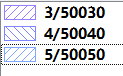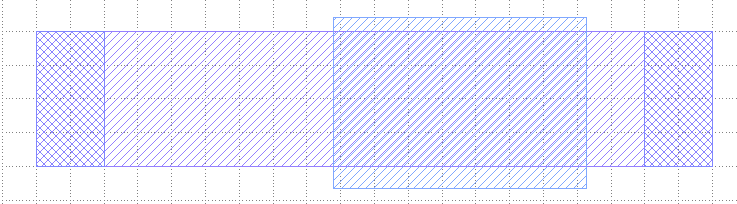Now in KLayout LVS when the device is extracted, all the pin layers will be stored in layer_geometry. layer_geometry records the shape of the pin, but not the position of the pin, the coordinates recorded in layer_geometry are relative, according to my observation, I think the lower left corner of layer_geometry is set is (0,0). For example, the Box of the left pin of resistor is lower left (11.06900,1.91100) upper right (12.06900,3.91100),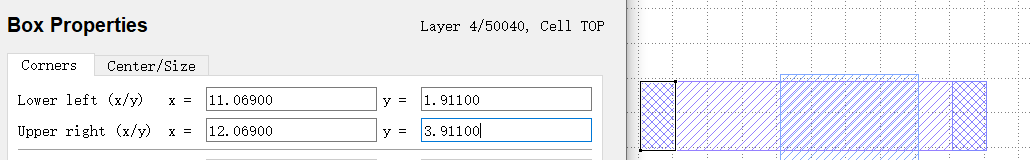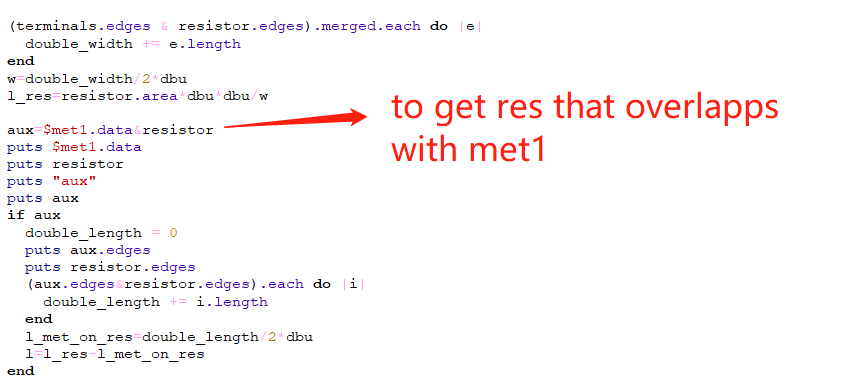Obviously, it is empty: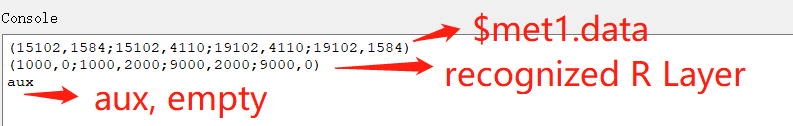The extracted polygons are reconverted with the lower left corner as the zero point, so they cannot be directly operated with the layers in the source file.

How can I achieve this? I have given the test case in the attachment. The length between the two pins is 8u, after receiving the influence of the aux layer, the calculated length should be 4u